# 1、拉格朗日插值

## 新建如下函数：

```function y=lagrange(x0,y0,x)
%拉格朗日插值函数
%n 个节点数据以数组 x0, y0 输入(注意 Matlat 的数组下标从1开始),
%m 个插值点以数组 x 输入,输出数组 y 为 m 个插值
n=length(x0);m=length(x);
for i=1:m
z=x(i);
s=0.0;
for k=1:n
p=1.0;
for j=1:n
if j~=k
p=p*(z-x0(j))/(x0(k)-x0(j));
end
end
s=p*y0(k)+s;
end
y(i)=s;
end```

## 应用实例：

```x0=1:1:20;
y0=x0.^2-20*x0-5;
x=1:0.1:20;
z=lagrange(x0,y0,x);
plot(x,z,‘:‘,x0,y0,‘ko‘);```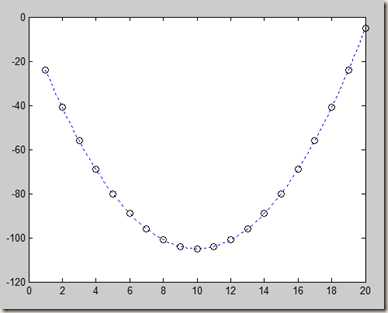# 2、分段线性插值

MATLAB现成的插值函数为interp1，其调用格式为：  yi= interp1(x,y,xi,‘method‘)

‘method‘：是最近项插值;                                                              ‘linear‘：线性插值;（默认）

‘spline‘：逐段3次样条插值; (下面的三次样条插值会用到)             ‘cubic‘：保凹凸性3次插值

‘pchip‘：分段三次Hermite 插值。

12，9，9，1，0，18 ，24，28，27，25，20，18，15，13，

```x=0:2:24;
y=[12,9,9,10,18,24,28,27,25,20,18,15,13];
x1=0:0.5:24;
y1=interp1(x,y,x1,‘linear‘);
plot(x,y,‘bo‘,x1,y1,‘r:‘);```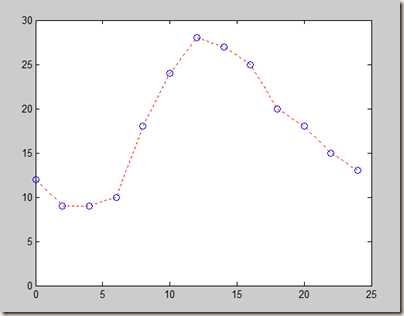# 3、埃尔米特插值

```function y = hermite( x0,y0,y1,x )
%埃尔米特插值多项式
%x0为点横坐标
%y0为函数值
%y1为导数值
%m个插值点用数组x输入
n=length(x0);m=length(x);
for k=1:m
yy=0.0;
for i=1:n
h=1.0;
a=0.0;
for j=1:n
if j~=i
h=h*((x(k)-x0(j))/(x0(i)-x0(j)))^2;
a=1/(x0(i)-x0(j))+a;
end
end
yy=yy+h*((x0(i)-x(k))*(2*a*y0(i)-y1(i))+y0(i));
end
y(k)=yy;
end```

# 4、样条插值

### 二次样条函数插值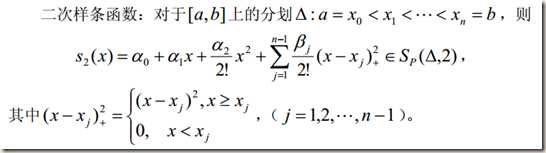（1）已知插值节点xi 和相应的函数值 yi (i = 0,1,…,n) 以及端点 x0 （或 xn ）处的导数值y‘0（或yn）

（2）已知插值节点xi 和相应的导数值 yi (i = 0,1,…,n) 以及端点 x0 （或 xn ）处的函数值y0 （或yn

### 三次样条函数插值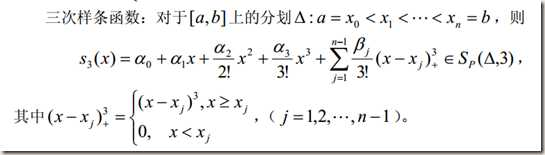（1）s‘3 (a) = y‘0 ,s‘3 (b) = yn 。由这种边界条件建立的样条插值函数称为 f(x) 的完备三次样条插值函数。

（2）s"3 (a) = y"0 ,s"3 (b) = y"3 。特别地 y"n = y"n = 0 时，称为自然边界条件。

（3）s‘3 ( a + 0) = s‘3 ( b − 0), s"3 (a + 0) = s"3 (b − 0) ， (这里要求 s3 (a + 0) =s3 (b − 0) )此条件称为周期条件。

### Matlab实现（三次样条插值）

Matlab中的函数：

1、y=interp1(x0,y0,x,`spline`);%(spline改成linear，则变成线性插值)

2、y=spline(x0,y0,xi);%这个是根据己知的x，y数据，用样条函数插值出xi处的值。即由x,y的值计算出xi对应的函数值。

3、pp=spline(x0,y0);%是由根据己知的x，y数据，求出它的样条函数表达式，不过该表达式不是用矩阵直接表示，要求点x`的值，要用函数y`=ppval(pp,x`);

4、pp=csape(x,y,‘变界类型‘,‘边界值conds‘);生成各种边界条件的三次样条插值. 其中,(x,y)为数据向量,边界类型可为:

‘complete‘：给定边界一阶导数,即默认的边界条件,Lagrange边界条件
‘not-a-knot‘：非扭结条件,不用给边界值.
‘periodic‘：周期性边界条件,不用给边界值.
‘second‘：给定边界二阶导数.
‘variational‘：自然样条(边界二阶导数为[0,0]

边界值conds可用1x2矩阵表示,矩阵元素取值为1,2,此时，使用命令
pp=csape(x0,y0_ext,conds)

conds(i)=j 的含义是给定端点 i j 阶导数， 即 conds 的第一个元素表示左边界的条

### 例子：

0  3      5   7     9  11  12  13  14  15
0 1.2 1.7 2.0 2.1 2.0 1.8 1.2 1.0 1.6

```clear,clc
x0=[0,3,5,7,9,11,12,13,14,15];
y0=[0,1.2,1.7,2.0,2.1,2.0,1.8,1.2,1.0,1.6];
t=0:0.05:15;
%拉格朗日插值函数
y1=lagrange(x0,y0,t);%调用编写的lagrange函数
dy1=(lagrange(x0,y0,0.0001)-lagrange(x0,y0,0))/0.0001%x=0处斜率
min1=min(lagrange(x0,y0,13:0.001:15))%13到15最小值
subplot(2,2,1);
plot(x0,y0,‘ro‘,t,y1);%画出曲线
title(‘拉格朗日插值函数‘);
%分段线性插值
y2=interp1(x0,y0,t,‘spline‘);%注意区分spline与linear
Y2=interp1(x0,y0,t);%默认linear
dy2=(interp1(x0,y0,0.0001,‘spline‘)-interp1(x0,y0,0,‘spline‘))/0.0001%x=0处斜率
min2=min(interp1(x0,y0,13:0.001:15,‘spline‘))%13到15最小值
subplot(2,2,2);
plot(t,y2,‘b‘,t,Y2,‘r‘,x0,y0,‘ro‘);%画出曲线
title(‘分段线性插值‘);
legend(‘边条‘,‘线性‘);%显示图形图例
%三次线条插值A
y3=spline(x0,y0,t);
dy3=(spline(x0,y0,0.0001)-spline(x0,y0,0))/0.0001%x=0处斜率
min3=min(spline(x0,y0,13:0.001:15))%13到15最小值
subplot(2,2,3);
plot(x0,y0,‘ro‘,t,y3);%画出曲线
title(‘三次线条插值A‘);
%三次线条插值B
pp1=csape(x0,y0);%默认的边界条件,即给定边界一阶导数
pp2=csape(x0,y0,‘second‘);%给定边界二阶导数
y4=ppval(pp1,t);
Y4=ppval(pp2,t);
dy4=(ppval(pp1,0.0001)-ppval(pp1,0))/0.0001%x=0处斜率
min4=min(ppval(pp1,13:0.001:15))%13到15最小值
subplot(2,2,4);
plot(t,y4,‘b‘,t,Y4,‘r‘,x0,y0,‘ro‘);%画出曲线
title(‘三次线条插值B‘);
legend(‘一阶‘,‘二阶‘);```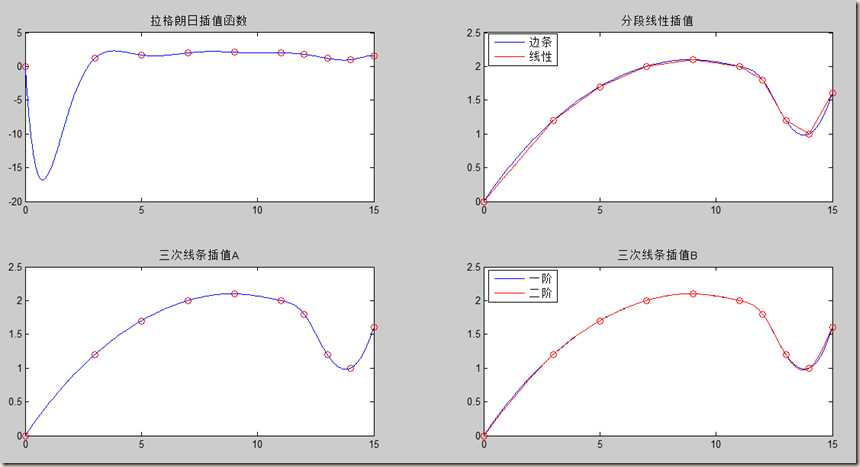```dy1 =

-55.2855

min1 =

0.9391

dy2 =

0.5023

min2 =

0.9828

dy3 =

0.5023

min3 =

0.9828

dy4 =

0.5007

min4 =

0.9851```

Matlab随笔之插值与拟合（上）

(0)
(0)

0条

© 2014 bubuko.com 版权所有 鲁ICP备09046678号-4